### 2.2. Notes on certain constructions with the verb "být"

#### 2.2.1. Verbonominal predicate vs. periphrastic passive

Constructions with a verbonominal predicate (see Section 2.1.3, "Copula "být" (verbonominal predicate)") and constructions with a reflexive passive cannot always be categorically distinguished: the short form of the deverbal adjective is morphologically identical with the periphrastic participle of the verb. Both types of construction (with a participle and with the short form of the deverbal adjective) are interpreted primarily as a periphrastic passive (see exception below). The difference between the meaning of "event " and the meaning of "resultant state" will be represented by the value of the grammateme of resultativeness (`resultative`; see Section 5.14, "The `resultative` grammateme (resultative aspect)"). Cf.:

• Dům byl.`PRED` zadlužený.`PAT` (=The house was mortgaged.)

Verbonominal predicate (cf. Fig. 8.20).

• Dům byl zadlužen.`PRED` [`t-lemma`=zadlužit; `sempos`=`v`] (=The house was mortgaged.)

Periphrastic passive with the meaning of "resultant state" (cf. Fig. 8.21).

• Dům byl rodinou zadlužen.`PRED` [`t-lemma`=zadlužit; `sempos`=`v`] (=The house was mortgaged.)

Periphrastic passive with the meaning of "event" (cf. Fig. 8.22).

!!! The values in the grammateme of resultativeness in constructions with a periphrastic passive have so far not been distinguished. The value `res0` is always entered in the grammateme of resultativeness in cases of a periphrastic passive.

Note: For purposes of annotation, the basis is the written utterance. The lack of distinction between the two types in spoken utterances cannot be reflected here (a speaker would say Výstava je otevřená (=The exhibition is open), but he would write Výstava je otevřena (=The exhibition is open)).

Exception: a construction expressing a resultant state which is the consequence of a reflexively conceived event. Only constructions expressing a resultant state which is the consequence of a reflexively conceived event are represented as verbonominal predicates: být + short form of a deverbal adjective (not as a periphrastic passive). These are cases where the construction really contains no evidence of a passively conceived event, where the sentence cannot be interpreted as involving a general Actor, because the surface subject is at the same time the deep level Actor of the expressed event directed at himself.

According to the rules introduced in Section 2, "The relation between a node's t-lemma and m-lemma and between its t-lemma and word form" the nodes representing short forms of adjectives have the t-lemma of the full-form adjective.

Cf:

• Prezident je.`PRED` přesvědčen.`PAT`, že vláda neplní své povinnosti. [ `t-lemma`=přesvědčený; `sempos`=`adj.denot`] (=The president is convinced that the government is not fulfilling its obligations.)

= Prezident se přesvědčil, že vláda neplní své povinnosti. (=The president convinced himself that the government is not fulfilling its obligatiions.)

The construction does not have the meaning: "někdo přesvědčil prezidenta, že .." (=someone convinced the president that...), but: "prezident přesvědčil sebe sama, že... " (=the president convinced himself that...) (cf. Fig. 8.23).

Further examples:

Vláda je.`PRED` připravena.`PAT` plnit své povinnosti. [ `t-lemma`= připravený; `sempos`=`adj.denot`] (=The government is prepared to fulfil its obligations.)

Premiér je.`PRED` unaven.`PAT` [`t-lemma`= unavený; `sempos`=`adj.denot`] (=The president is tired now.)

Pavel ještě není.`PRED` oblečen.`PAT` [`t-lemma`= oblečený; `sempos`=`adj.denot`] (=Paul is not dressed yet.)

!!! The choice between verbonominal predicate and periphrastic passive, or between short-form adjective and passive participle was made only at the tectogrammatical level. At the morphological level the expressions přesvědčen (=convinced), unaven (=tired), připraven (=prepared) etc. are always evaluated as verb forms.

The choice between short-form adjective and passive participle concerns also positions other than after the verb být; see Section 5.1.2, "Dependent participial constructions".

Figure 8.20. Verbonominal predicate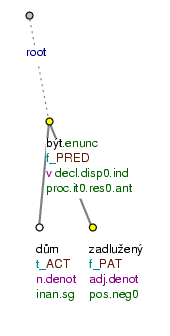Dům byl zadlužený. (=lit. (The) house was mortgaged.)

Figure 8.21. Periphrastic passive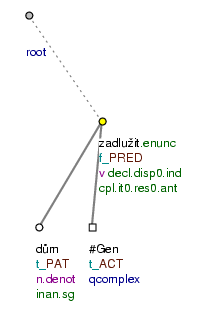Dům byl zadlužen. (=lit. (The) house was mortgaged.)

Figure 8.22. Periphrastic passive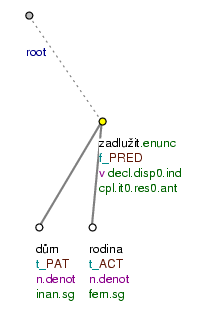Dům byl rodinou zadlužen. (=lit. (The) house was by_the_family mortgaged.)

Figure 8.23. Verbonominal predicate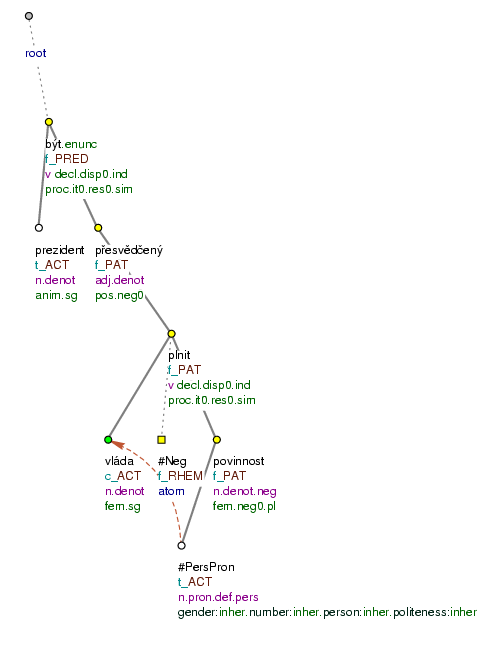Prezident je přesvědčen, že vláda neplní své povinnosti. (=lit. (The) president is convinced that (the) government is_not_fulfilling its obligations.)

#### 2.2.2. Constructions with the infinitive

In constructions with the verb být the infinitive can be Actor, Patient or obligatory adjunct.

The infinitive as Actor. Constructions in which the infinitive in the construction with the verb být (=to be) is the Actor occur in combinations with all types of the verb být (=to be), with existential, copula and substitute "být".

• The construction: existential "být" + infinitive in the position of Actor expresses the duration of some state, the existence of some possibility.

Examples:

Je co číst.`ACT` (=There is something to read.) Fig. 8.24

Je komu pomáhat.`ACT` (=Somebody needs help.)

Je pořád co dělat.`ACT` (=There is always something to do.)

• In constructions with the copula "být", the infinitive can be the Actor in the verbonominal predicate, but also the Patient. The infinitive takes the position of Actor especially in the sub-type of quasi-modal verbs (see Section 2.1.3, "Copula "být" (verbonominal predicate)").

Examples:

Je mu trapné odejít.`ACT` (=He is embarrassed to leave.) Fig. 8.25

Je marné o tom hovořit.`ACT` (=It is pointless to discuss it.)

Je možné studovat.`ACT` v zahraničí. (=It is possible to study abroad.)

• In particular, the infinitive regularly occurs as an Actor in constructions být + a modal adverb represented as a node with the functor `CPHR` (see Section 2.1.3, "Copula "být" (verbonominal predicate)").

Example:

Není nutno zdravit.`ACT` (=It is not necessary to give a greeting.)

• The infinitive may also be an Actor in constructions with substitute "být".

Example:

Teď je pozdě litovat.`ACT` (=It is too late for regrets now.) Fig. 8.26

A specific construction in which the infinitive takes the position of Actor is the construction být + infinitive with verbs of sensual perception and recognition: slyšet (=to hear), vidět (=to see), cítit (=to feel/sense/smell), poznat (=to recognise), rozumět (=to understand), the type "Je vidět Sněžku" (=Sněžka can be seen) (see Section 2.2.4, "The construction "Je vidět Sněžku/Sněžka"").

Infinitive as Patient. The non-verbal (non-copula) constituent of a verbonominal predicate (see Section 2.1.3, "Copula "být" (verbonominal predicate)") can also be expressed by an infinitive.

Examples:

Končit není umřít.`PAT` (=To finish is not to die.) Fig. 8.26

Je vidět.`PAT` , že se dobře bavíte. (=Clearly, you are having a good time.)

Je cítit.`PAT` síra. (=There is a smell of sulphur.)

Je vidět.`PAT` Sněžka. (=Sněžka can be seen.)

See also Section 2.2.4, "The construction "Je vidět Sněžku/Sněžka"" (construction "Je vidět Sněžku. (=Sněžka can be seen.)").

Infinitive as adjunct. In constructions with substitute "být" the infinitive can also take the position of an adjunct, most frequently as `INTT`.

Example:

Byli jsme to obhlížet.`INTT` (=We have been to inspect it.) Fig. 8.28

Figure 8.24. Infinitive as Actor in a construction with the verb "být"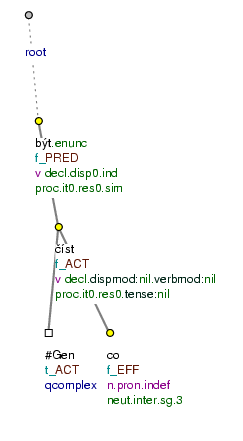Je co číst. (=lit. Is what to_read.)

Figure 8.25. Infinitive as Actor in a construction with the verb "být"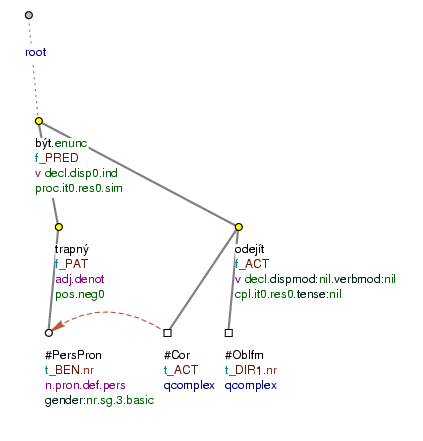Je mu trapné odejít. (=lit. Is him embarrassing to_leave.)

Figure 8.26. Infinitive as Actor in a construction with the verb "být"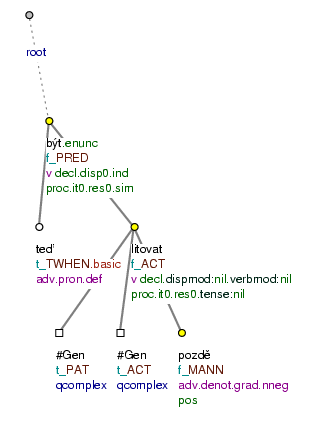Teď je pozdě litovat. (=lit. Now is late to_regret)

Figure 8.27. Infinitive as Patient in a constructiion with the verb "být"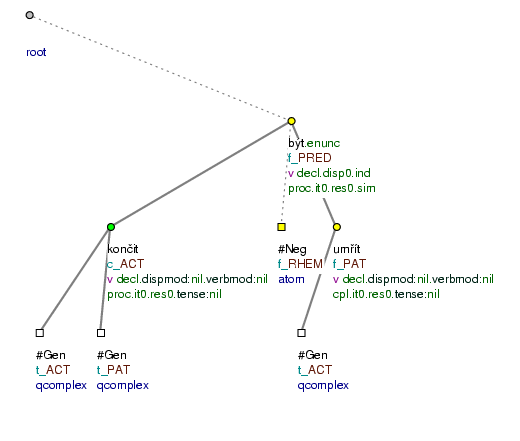Končit není umřít. (=lit. To_finish is_not to_die.)

Figure 8.28. Infinitive as a free modification in a construction with the verb "být"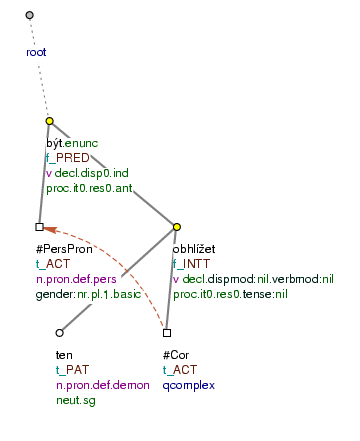Byli jsme to obhlížet. (=lit. (We) have_been AUX it to_inspect)

#### 2.2.3. Constructions with numerical expressions

The representation of constructions with numerical expressions varies according to whether the numeral is nominal, adjectival or adverbial in nature (on this, see Section 10, "Numbers and numerals" and Section 2, "Semantic parts of speech"):

• "být" + numeral or nominal and adjectival expressions.

Combinations of numerals and nominal and adjectival numerical expressions (semantic nouns or adjectives) with the verb být (=to be) are treated as verbonominal predicates (see Section 2.1.3, "Copula "být" (verbonominal predicate)"). A node for the governing noun is not added to an adjectival numeral in the position of Patient (see also Section 10.1.1, "Numerals with the role of an attribute (`RSTR`)").

Examples:

Je to.`ACT` třetina.`PAT` dortu. (=It is a third of the cake.) Fig. 8.29

To.`ACT` je kousek.`PAT` dortu. (=This is a piece of cake.)

Petr.`ACT` byl první.`PAT` (=Peter was first.)

Oni.`ACT` jsou tři.`PAT` (=There are three of them.)

Bylo to.`ACT` půl.`PAT` kilometru. (=It was half a kilometre.)

Je to.`ACT` třicet metrů.`PAT` (=It is thirty metres.)

Cena.`ACT` je třicet korun.`PAT` (=The price is thirty crowns.)

We are aware that in the case of the copula "být" the position of Patient carries a variety of meanings, especially in constructions with být + a numerical expression: identity (To je třetina dortu. (=That is a third of the cake.)), quantification (Cena je 30 Kč. (=The price is 30 Kč), Chlapců je pět. (=There are five boys.)), qualification (Petr byl první. (=Peter was first.)). The boundary between the respective types of meaning is not always clear-cut. The type of meaning of the verbonominal predicate is frequently determined only by the context - and for this reason only a semantically ambiguous Patient stands in the position of the non-verbal constituent.

• "být" + numeral or adverbial numerical expression.

In combinations of numerals and numerical expressions of an adverbial nature (semantic adverbs) with the verb být (=to be) the verb "být" is substitute (see Section 2.1.2, "Substitute "být""), or existential (see Section 2.1.1, "Existential "být""). Here, the numerical expression is an adjunct.

Examples:

Kurs.`ACT` byl dvakrát.`THO` (=The course took place twice.) Fig. 8.31

To.`ACT` je kousek.`EXT` od města. (=That is a short distance from the town.)

NB! The numerals hodně (=a lot), dost (=enough), moc (=too much), málo (=too little), mnoho (=many/much) (and their gradations více (=more), méně (=less)) in combinations with the verb být (=to be) are syntactic (and also semantic) adjectives (further on this, see Section 10.2.2, "Numeral expressions "hodně", "více", "dost", "moc", "málo", "méně", "stejně", "plno""). In constructions with the verb být, like adjectives, they then have the nature of a Patient; for example:

To.`ACT` je hodně.`PAT` (=That is a lot.) Fig. 8.30

To.`ACT` je moc.`PAT` (=That is too much.)

Problémů.`ACT` je více.`PAT` (=There are more problems.)

Děvčat.`ACT` je málo.`PAT` (=There are too few girls.)

Chlapců.`ACT` je ještě méně.`PAT` (=There are even fewer boys.)

To.`ACT` je o důvod víc.`PAT` (=That is a further reason.)

Figure 8.29. "Být" + a nominal or adjectival numeral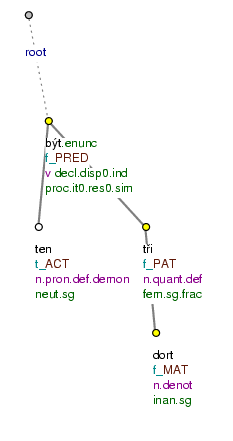Je to třetina dortu. (=lit. Is that a_third (of) (the) cake.)

Figure 8.30. "Být " + a nominal or adjectival numeral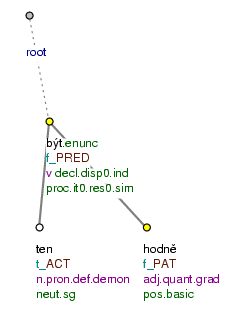To je hodně. (=lit. That is a_lot.)

Figure 8.31. "Být" + adverbial numeral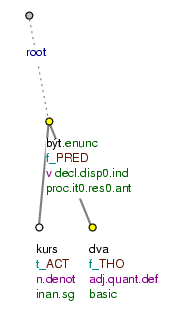Kurs byl dvakrát. (=lit. Course was twice.)

#### 2.2.4. The construction "Je vidět Sněžku/Sněžka"

A particular type of construction with the verb být (=to be) is one in which the verb být is associated with infinitives of verbs of sensual perception and recognition: slyšet (=to hear), vidět (=to see), cítit (=to sense, feel, smell), poznat (=to recognise), rozumět (=to understand). In these constructions the object of perception or recognition may be expressed either by the nominative of the noun or by the accusative; cf.:

• Je vidět Sněžka. (=Sněžka.NOM can be seen.)

• Je vidět Sněžku. (=Sněžka.ACC can be seen.)

• Je cítit síra. (=There is a smell of sulphur.NOM)

• Je cítit síru. (=There is a smell of sulphur.ACC)

A semantic distinction is made between the constructions and they are represented differently.

The construction "Je vidět Sněžka ". (=Sněžka can be seen.) The construction být + infinitive of a verb of sensual perception or recognition + the nominative of the noun is interpreted according to the model:

• Je vidět Sněžka. (=Sněžka can be seen.) = Sněžka je viditelná. (=Sněžka is visible.)

It is considered that the object expressed in the nominative can be perceived or recognised.

Here, the expression být + infinitive is treated as a verbonominal predicate (see Section 2.1.3, "Copula "být" (verbonominal predicate)"). The noun in the nominative is the Actor of the verb and the infinitive is its Patient.

Examples:

Je vidět.`PAT` Sněžka.`ACT` (=Sněžka is visible.) Fig. 8.32

Je slyšet.`PAT` hudba.`ACT` (=Music can be heard.)

Je znát.`PAT` změna.`ACT` (=A change is noticeable.)

The construction "Je vidět Sněžku". (=Sněžka can be seen.) The construction být + infinitive of a verb of sensual perception or recognition + accusative of the noun is a specific construction, similar to the construction with a predicate lze (=it is possible) (cf. Fig. 8.34). In this construction, by contrast with a construction with an object of perception or recognition expressed in the nominative, a certain meaning of modality is present, and at the same time one can imagine a subject which perceives or recognises. Constructions with an object of perception or recognition are therefore interpreted according to the model:

• Je vidět Sněžku. (=Sněžka.ACC can be seen.) = Anybody can see/it is possible for anybody to see Sněžka.

The verb být (=to be) is assigned a special valency frame in these constructions: `ACT`(.f). In these constructions the infinitive is treated as the Actor of the verb být (=to be). The subject of the perception or recognition is represented as a free benefActor (`BEN`), which, unless it is explicit, is added to the tectogrammatical tree only for purposes of representing the grammatical co-referential relationships (see Section 2.4, "Control").

Examples:

Je vidět.`ACT` Sněžku. (=Sněžka can be seen.) Fig. 8.33

Je slyšet.`ACT` hudbu. (=Music can be heard.)

Je znát.`ACT` změnu. (=A change is noticeable.)

A construction with an object of perception or recognition in the accusative is treated as basic, and it is the model for the representation of examples where the case of the noun cannot be determined, where the object of perception or recognition is not expressed at all, and also where the noun is replaced by a dependent clause or an adverbial.

Examples:

Bylo slyšet.`ACT` střílení. (=Firing could be heard.) Fig. 8.35

Přednášejícímu nebylo rozumět.`ACT` (=The lecturer could not be understood.) Fig. 8.36

Je vidět.`ACT` , že máš pravdu. (=It can be seen that you are right.) Fig. 8.37

Odsud je vidět.`ACT` až za hranice. (=From here one can see beyond the frontier.) Fig. 8.38

Figure 8.32. The construction "Je vidět Sněžka"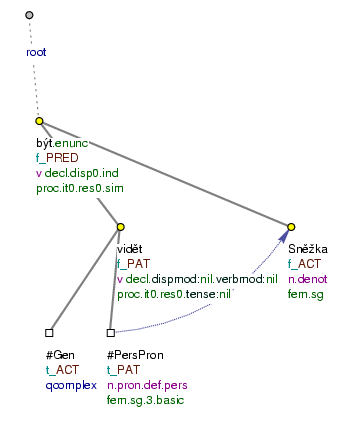Je vidět Sněžka. (=lit. Is to_see Sněžka.)

Figure 8.33. The construction "Je vidět Sněžku"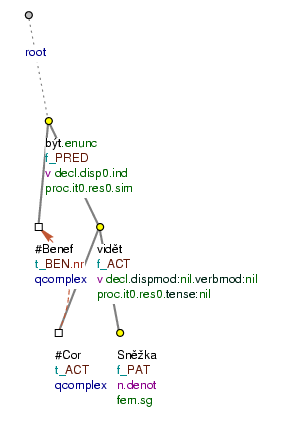Je vidět Sněžku. (=lit. Is to_see Sněžka.ACC)

Figure 8.34. The construction with a predicate "lze"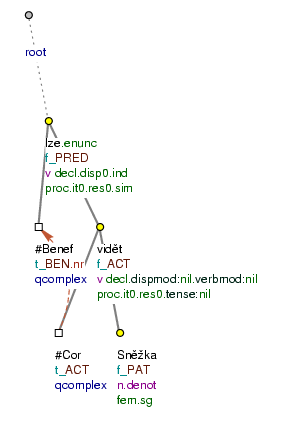Lze vidět Sněžku. (=lit. It_is_possible to_see Sněžka.ACC)

Figure 8.35. The construction "Je vidět Sněžku"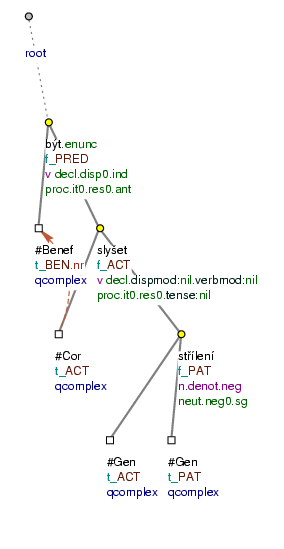Bylo slyšet střílení. (=lit. Was to_hear firing)

Figure 8.36. The construction "Je vidět Sněžku"Přednášejícímu nebylo rozumět. (=lit. (The) lecturer.DAT was_not to_understand.)

Figure 8.37. The construction "Je vidět Sněžku"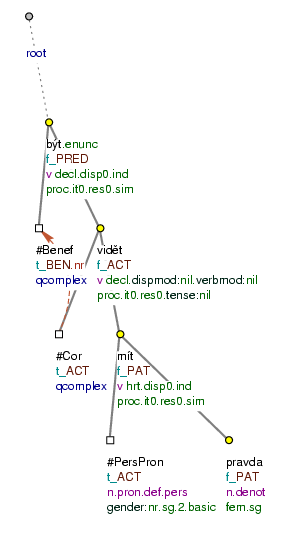Je vidět, že máš pravdu. (=lit. Is to_see that (you) are right.)

Figure 8.38. The construction "Je vidět Sněžku"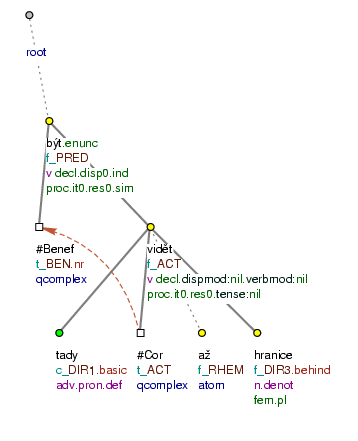Odsud je vidět až za hranice. (=lit. From_here is to_see - beyond (the) frontier.)